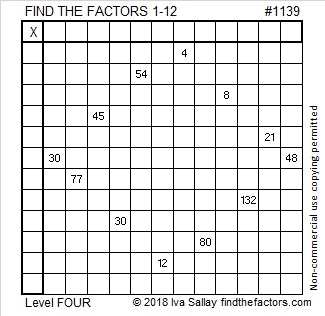# 1139 and Level 4

The common factors of 30 and 48 are 1, 2, 3, and 6. The rules of a Find the Factors 1 – 12 puzzle require that only numbers from 1 to 12 go in either the first column or the top row. Each number can be used only once in each place. Which common factor of 30 and 48 must be chosen?Print the puzzles or type the solution in this excel file: 12 factors 1134-1147

Here are some facts about the number 1139:

• 1139 is a composite number.
• Prime factorization: 1139 = 17 × 67
• The exponents in the prime factorization are 1 and 1. Adding one to each and multiplying we get (1 + 1)(1 + 1) = 2 × 2 = 4. Therefore 1139 has exactly 4 factors.
• Factors of 1139: 1, 17, 67, 1139
• Factor pairs: 1139 = 1 × 1139 or 17 × 67
• 1139 has no square factors that allow its square root to be simplified. √1139 ≈ 33.749071139 is the sum of seven/eleven consecutive primes:
149 + 151 + 157 + 163 + 167 + 173 + 179 = 1139
79 + 83 + 89 + 97 + 101 + 103 + 107 + 109 + 113 + 127 + 131 = 1139

1139 is the hypotenuse of a Pythagorean triple:
536-1005-1139 which is (8-15-17) times 67

This site uses Akismet to reduce spam. Learn how your comment data is processed.